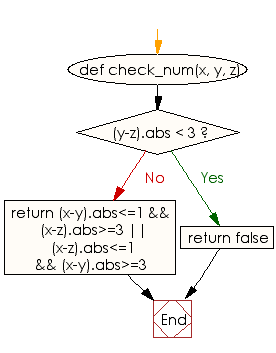﻿ Ruby Basic exercises: Check three given integers x, y, z and return true if one of y or z is close while the other is far - w3resource# Ruby Basic Exercises: Check three given integers x, y, z and return true if one of y or z is close while the other is far

## Ruby Basic: Exercise-54 with Solution

Write a Ruby program to check three given integers x, y, z and return true if one of y or z is close (differing from a by at most 1), while the other is far, differing from both other values by 3 or more.

Ruby Code:

``````def check_num(x, y, z)
if (y-z).abs < 3
return false
end
return (x-y).abs<=1 && (x-z).abs>=3 || (x-z).abs<=1 && (x-y).abs>=3
end
print check_num(2, 3, 11),"\n"
print check_num(2, 3, 4),"\n"
print check_num(5, 2, 4)
``````

Output:

```true
false
false
```

Flowchart:Ruby Code Editor: